## Sunday, July 28, 2013

### Round function in MDX

SQL Server 2005-2012

In most cases you will want to perform a round-operation of your MDX query results by applying a format string. Alternatively, you may want to use client functionality (for example formatting capabilities in Excel) to show the results in the required format.

However, there are some cases, in which you may want the cube to return a rounded result directly. This could be the case for a financial cube where you want to have exactly the same type of rounding as in the ERP software behind.

First let’s look at the formatting of a measure. The following simple query shows how to format a given value to a fixed number of decimal places:

with member testvalue as 0.5, format_string='#,##0'
select [Measures].[testvalue] on 0 from [Adventure Works]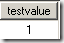The method used for rounding here is often referred to as ‘round half up’ because half way values are always rounded up. For example, if you round 0.35 to one digit after the decimal point you would get 0.4.

Since we used format_string the formatting is applied to the value as an additional property. For example

with
member testvalue as 0.57372843213
member testvalueformatted as testvalue, format_string = '#,##0.00'
member derivedvalue as testvalueformatted, format_string='#,##0.000000'

select {
[Measures].[testvalue]
, [Measures].[testvalueformatted]
, [Measures].[derivedvalue]
}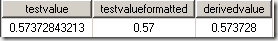In this example we created a formatted value with a precision of two digits. Then we created a new measure based on the formatted one but this time with 6 digits. What you can see, is that no information is lost because of the formatting, it’s just for display.

I wrote about more complex formatting capabilties here, here and here. And there is another post explaining how to make sure that the rounded sum corresponds to the rounded detail rows.

Now, let’s assume you want to perform the rounding directly in MDX. One option to do so is the VBA round function. The function takes two parameters:

1. The value that you want to round
2. The number of digits after the decimal point

For example, to round a given value to 2 digits after the decimal point you could write something like this:

with member testvalue as VBA!round(0.57372843213,2)
select { [Measures].[testvalue] }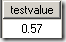However, the reason I’m writing this blog post is a warning:

Different rounding-methods exist and are in practical use and therefore two implementations of a rounding function do not necessarily get to the same result.

In fact, the method used be VBA!round(…) is actually ‘round half to even’, not ‘round half up’ (as with our format_string). The difference can be clearly seen if you perform a rounding operation to the integer value. In this case 0.5 is rounded to the nearest even integer value which is 0 (not 1). This method is more statistically balanced because it doesn’t prefer half way values and is therefore widely used in bookkeeping. You can see the same effect in Excel. The worksheet round function uses ‘round half up’ while the build-in VBA function uses ‘round half to even’. Here is the corresponding effect in MDX:

with
member val1 as VBA!round(0.5,0)
member val2 as VBA!round(1.5,0)

select { [Measures].[val1], [Measures].[val2] }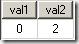• 0.5 rounds to 0
• 1.5 rounds to 2

You can find a lot more about different rounding methods on Wikipedia. So, if the requirement is to show rounded values (in the cube, on the report or wherever) you should always be sure to understand the rounding method.

To get back to the original rounding requirement in MDX you could perform a rounding operation (round half up) for the value x by using this formula: Int( x + sgn(x)*0.5)

This is shown in the following MDX query:

with
member x as 0.5
member x_rounded as VBA!Int(x +VBA!Sgn(x)*0.5)

select { x, x_rounded }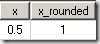And of course, if you need to round to a giving precision you could multiply/divide the value appropriately, for example like this

with
member x as 0.2353
member x_rounded as VBA!Int(100*x +VBA!Sgn(x)*0.5)/100

select { x, x_rounded }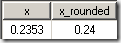If you have a lot of such operations I would recommend to put the rounding-functions in an Assembly. And again, best choice is to round by format, not by calculation, so the procedure above is only recommended if you really need to return a properly (or specifically) rounded result from the cube.

## Saturday, July 20, 2013

### Considerations for the distribution key for distributed tables on PDW (part 2)

PDW v1/PDW 2012

In my last post I started with two important considerations for the distribution key:

• Skew (data being equally distributed)
• Distribution compatibility (table joins for most typical queries are supported in an optimal way)

So, here are the remaining two considerations, I’d like to present here:

Consideration 3: Aggregation compatible queries

Expected aggregations also play a role when choosing a good distribution key. There is an excellent post by Stephan Köppen about aggregation compatible queries. As ‘aggregation’ says, we’re talking queries that do a group by (like many DWH queries do). As long as the distribution key is present in the group by field list, PDW can be sure that each compute node can do the aggregation on its on (each aggregation group is on a single compute node). For example, in the following example we have a sales table that is distributed on the article and we’re running a query that groups sales by article: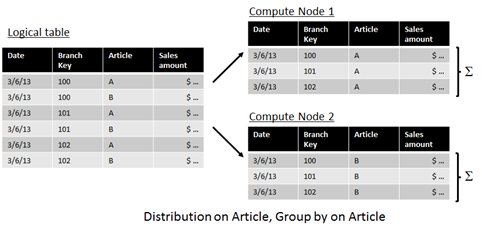As you can see, distribution on article guarantees that all the rows for article A are getting to the same compute node. Therefore a group-by-operation on article A can be performed on this node. The same is true for article B (may be hashed to the same compute node as article A, but the point is that all rows of article B also sit on the same compute node). Please note that this is just an example. Depending on the hash function article B may be on the same node as article A but the point is that all rows for each article are on the same compute node.

Now let’s assume we’re still distributing on article but now we’re doing a group by on the branch: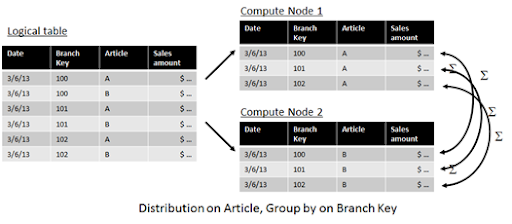In this case, rows for the same branch (may be) spreaded over several compute nodes. Therefore it is necessary for the PDW to move data between the nodes in order to perform the group by operation.

Again, I’d like to refer the Stephan’s post regarding the way PDW handles aggregations. Even if a single node can handle the “group by” on its own it may still be necessary to “blend” the results on the compute node. For example, if we query the example above on article and branch, each node would deliver three aggregated values which then have to be put together in order to get the final result set.

So, as a conclusion we can say that a query is aggregation compatible if the distribution key is present in the group-by field list.

Consideration 4: Query behavior and typical workload

As explained in considerations 2 and 3 we have to consider the expected workload in order to find a good distribution key (and table layout, i.e. merging two tables into one, choosing replicated tables etc.). And this is also true for consideration 1. While usually we want to distribute our data "equally” on all of the compute nodes (no skew), there may be query situations that may be supported by a different distribution strategy. For example, in a call center we want the call center agent to access aggregated values for a single customer almost instantly when the customer is routed to the call center agent’s desk. In this case, the group-by will contain an identification for the customer (customer number, phone number etc.). Now if the data for this customer is distributed over multiple compute nodes, PDW has to collect this data on the control node before it can be routed back to the caller. If the data is only one compute node, this node can immediately start a so called “return operation” routing the data directly to the query client (no action needed on the control node). The difference in query time may not be that much, but imagine you have a very large call center with a lot of calls getting in each minute. So instead of few queries scanning a large amount of data, we now have lots of queries that are rather simple and only accessing a small fraction of total data. Distributing single queries on multiple machines wouldn’t give a benefit here, but distributing the query workload on multiple machines would help (much like a classical SQL Server cluster). And in this scenario we wouldn’t even care much about possible skew in the data.

So, the query behavior plays an important role when deciding for a good distribution key.

Putting it all together

When reading the considerations from above, one might think that deciding for the best distribution key seems is a very difficult process. Since it depends on the query behavior, you can’t prepare yourself for every possible query. So what shall we do?

Of course, we want to get the best possible performance out of the PDW, but keep in mind:

• Even with a “non-optimal” distribution key, PDW will still be extremely fast. Moving data between the nodes is what the PDW is designed for. So doing a shuffle move operation is not necessarily a bad thing.
• The decision for the distribution key is not a decision to last for ever. In fact you can easily redistribute the table (CTAS operation) based on another distribution key, if you think that the other key is better
• When talking about query performance, statistics are also very important for the query optimizer to generate a good query plan. Make sure your statistics are always up to date.

In most cases you’re best advised to look for a column with a high number of distinct values. If you know that you frequently need to join this to another distributed table, take this into account (join key is a candidate for the distribution key if it has a high enough cardinality). In some rare cases you may want to create pre-aggregated tables (to have more queries being aggregation compatible) or keep multiple versions of the same distributed table with different distribution keys. But again, in most cases finding a good distribution is not rocket science and you will be surprised how well the PDW works even with distribution keys being not optimal.

## Sunday, July 14, 2013

### Considerations for the distribution key for distributed tables on PDW (part 1)

PDW v1/PDW 2012

In a previous post I briefly touched the question how to choose a good distribution key for a PDW distributed table. And also in this post I promised to get back to this topic in more detail later. The distribution key is a data base table column used to determine the distribution on the compute nodes. This is shown in the following illustration from my previous post, where 6 fact rows are distributed on two compute nodes based on the values in the date column.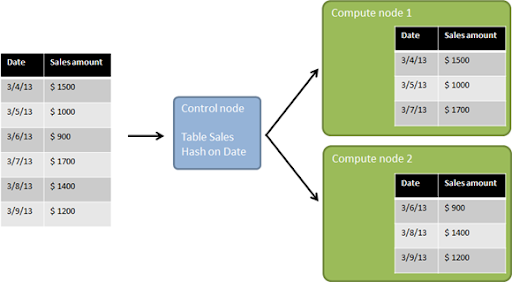The table DDL statement would look somewhat like this:

CREATE TABLE [Sales].[dbo].[FactSales]
(
[Date] date NOT NULL,
[Sales amount] MONEY NOT NULL
)
WITH (DISTRIBUTION = HASH ([Date]));

The values in the distribution key column (Date column in my example) are mapped to the distribution using a hash function. The hash function is not a simple round-robin, but takes into account the number of compute nodes and the column’s data type (for example int is hashed differently compared to bigint) and can (currently) not being queried directly. If a scale unit is added to the PDW (therefore bringing more compute nodes into the appliance) the existing data is redistributed and the internal hash function then takes the new nodes into account (different hash values).

One more thing to notice before we look at considerations for the distribution key column is that the distribution is not only happening on the compute node level, but the data is also distributed within each of the compute node. Each logical table of the data model becomes 8 tables (postfixed with letter a to h) on each of the compute nodes to support different file groups. Don’t confuse this with table partitions. Table partitioning works on top of this. So, assuming you have 10 compute nodes, a single distributed table (logical database view) with 10 partitions becomes 800 physical partitions (each of the 8 distributed tables per compute node with 10 partitions giving 80 partitions, multiplied by the number of compute nodes). Fortunately enough, PDW takes care about all that fully automatically. You just have to create those 10 partitions on the original table. PDW does the magic behind.

So, after this short introduction, let’s get back to the question, what we need to consider in order to find a good distribution key. Please note that I’m saying ‘considerations’, not recommendations or guidelines. The reason is that depending on your data and your typical query work load, you should consider the topics below to find a good solution for yourself.

Consideration 1.: Skew

We’re using the term skew here to describe the situation where some distributions contain much more or much less data than the average of the distributions. If typical queries involve a large number of rows, the power of the PDW depends on the ability to parallelize the query to its nodes. In the worst case, if all the data sits on only one compute node, PDW behaves much like an SMP machine. Depending on the structure of the query (see considerations 2 and 3), the “slowest” compute node sets the time for the query to complete. So, we want all compute nodes to contain about the same amount of data to get the most out of the PDW (not much skew).

You may notice however that I mentioned the query behavior (large number of rows involved). This topic is extremely important and I’m getting back to this with Consideration 4.

Usually, to prevent skew, you would look for an equally distributed column with many distinct values. In my example from above, I used Date as the distribution column. Is this a good idea? Assuming that you have different buying behavior depending on the season, it might lead to a significant skew. So date might not be a good column to base your distribution on. Usually good candidates are fields like order number, transaction number, any kind of sequential counters etc.

In order to show the effect, I’m using a table FactSales with about 9 million rows on a PDW with 2 compute nodes (giving 16 distributions). First, I’m going to distribute the data based on the order number. In order to see how the distribution works, we’re using the PDW_SHOWSPACEDUSED function:

DBCC PDW_SHOWSPACEUSED("dbo.FactSales")

Here is the result: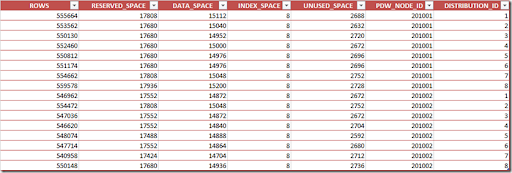The compute node can be found in the next to last column (PDW_NODE_ID). The distribution number on the compute node itself is shown in the last column (1-8 per compute node). As you can see, the distribution (rows, first column) is quite balanced. Here’s how it looks when plotted over the distributions: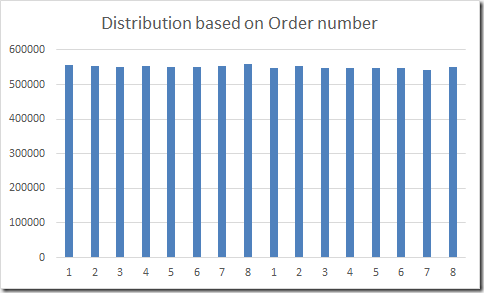Next, let’s distribute the same data based on the product. Since some products are sold more often than others, we expect some skew to occur. And for the (real life) data I used here, this is true as you can see from the following visualization: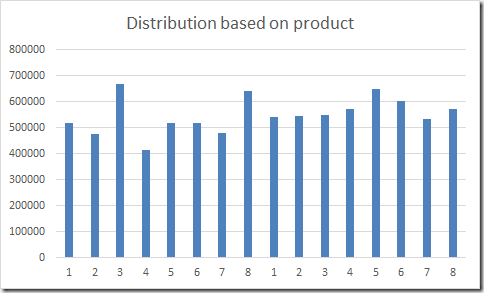My data also contains the line number (per order). I expect this to be a very bad distribution key because I don’t have many orders with more than 30 order lines. So, here is the distribution per line number: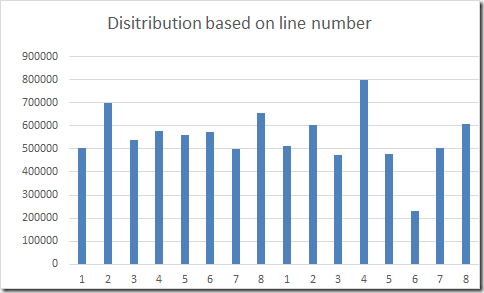In this case, you can see significant skewing effect. The number of rows in distribution 4 (node 2) is 4 times the number of distribution 6. I even expected the distribution to be worse, since the distribution of the order line column has a significant skew. Here’s a histogram of the order line column: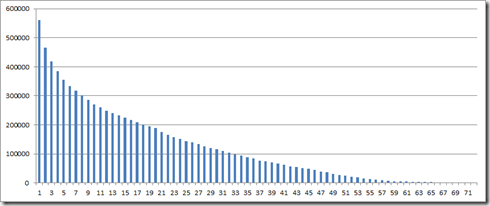So, you see, that the build-in hash function of the PDW does a great job. However, if you want to reduce skew in order to leverage the full parallel power of the PDW for queries involving a large amount of rows, make sure to choose a column with equally distributed values. Usually you’re looking for a column with a lot of distinct values. As you can imagine, if your distribution key column has less distinct values than the number of distributions, you’ll end up with some distributions having no data at all (bad choice).

Consideration 2: Distribution compatible queries (joins)

For parallelization, our goal is that each node can work on the query independently. For more complex queries however, it becomes necessary to shuffle data between the nodes. When looking at the distribution compatibility of queries, we try to optimize the need for shuffling data between the nodes. In general, a query is distribution compatible if the data resulting from right side of the join sits on the same node as the data resulting from the left side of the join. For a normal SMP machine this is always true, but for distributed tables, if the distribution on the left and right side of the join is based on different criteria, it’s getting more complicated.

First, let’s take a look at a star join, where the join destinations are replicated tables.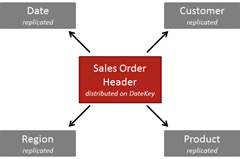In this case we’re joining a Sales Order Header table to it’s dimension. All dimensions are replicated tables here, so no matter on which column we’re distributing the Sales Order Header table, we’re always sure to find the matching data on the same node (as the data is available on every node, distribution of the Sales Order Table doesn’t matter in this case).

Now, let’s assume we’re joining the Sales Order Header table (distributed on DateKey) with its Sales Order Detail rows (distributed on DateKey). The join column is the OrderNumber. Now for a given row of the Sales Order Header table the PDW cannot be sure that corresponding rows of the Sales Order Detail table are located on the same node as they’re distributed based on the DateKey, not on the OrderNumber. This causes shuffle move operations in order to get both sides being aligned on their join condition.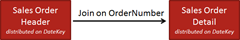Anyway, in this practical case, we know that the rows are on the same machine as each order number refers to an order of a specific date. So one order cannot have two different dates. But this is implicit knowledge that is not available to the query optimizer in order to find a good query plan.

So, in order to get a distribution compatible join between these two tables, we could either

• distribute both tables on the OrderNumber (which makes sense, since both tables will frequently being queried using this column as a join criteria)
or
• Include the DateKey in the column-list for the join (this is an option for views or for the Data Source View of an SSAS database for example)

By including the distribution key in the column list for the join we’re letting PDW know, that the grain of the join is truly finer than the distribution, so it can process each the join-operation on each node separately.

But wait, we talked about considerations for the distribution key of the data base table and now we’re looking at queries and joins. But this is right, the decision for the distribution key depends a lot on the expected work load and on the question which queries you like to support best with your distribution architecture. I’m getting back to this in consideration 4 later.

To be continued with the next post.